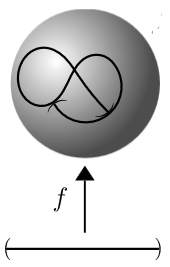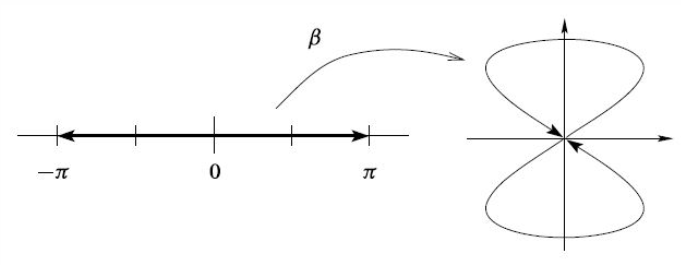# nLab immersion of smooth manifolds

Contents

### Context

#### Differential geometry

synthetic differential geometry

Introductions

from point-set topology to differentiable manifolds

Differentials

V-manifolds

smooth space

Tangency

The magic algebraic facts

Theorems

Axiomatics

cohesion

• (shape modality $\dashv$ flat modality $\dashv$ sharp modality)

$(\esh \dashv \flat \dashv \sharp )$

• dR-shape modality$\dashv$ dR-flat modality

$\esh_{dR} \dashv \flat_{dR}$

infinitesimal cohesion

tangent cohesion

differential cohesion

singular cohesion

$\array{ && id &\dashv& id \\ && \vee && \vee \\ &\stackrel{fermionic}{}& \rightrightarrows &\dashv& \rightsquigarrow & \stackrel{bosonic}{} \\ && \bot && \bot \\ &\stackrel{bosonic}{} & \rightsquigarrow &\dashv& \mathrm{R}\!\!\mathrm{h} & \stackrel{rheonomic}{} \\ && \vee && \vee \\ &\stackrel{reduced}{} & \Re &\dashv& \Im & \stackrel{infinitesimal}{} \\ && \bot && \bot \\ &\stackrel{infinitesimal}{}& \Im &\dashv& \& & \stackrel{\text{étale}}{} \\ && \vee && \vee \\ &\stackrel{cohesive}{}& \esh &\dashv& \flat & \stackrel{discrete}{} \\ && \bot && \bot \\ &\stackrel{discrete}{}& \flat &\dashv& \sharp & \stackrel{continuous}{} \\ && \vee && \vee \\ && \emptyset &\dashv& \ast }$

Models

Lie theory, ∞-Lie theory

differential equations, variational calculus

Chern-Weil theory, ∞-Chern-Weil theory

Cartan geometry (super, higher)

# Contents

## Definition

Let $f \colon X \to Y$ be a differentiable function between smooth manifolds (or just differentiable manifolds) of finite dimension.

We denote by $T X$, $T Y$ the tangent bundles and by $(-)\times_Y (-)$ the fiber product of differentiable functions into $Y$. In particular, $f^\ast T Y \,=\, X \times_Y T Y$ is the pullback bundle of $T Y$ along $f$ to a real vector bundle over $X$.

###### Definition

The differentiable function $f : X \to Y$ is called an immersion precisely if the canonical map

$T X \longrightarrow X \times_Y T Y \eqqcolon f^* T Y$

is a monomorphism.

This map is the one induced from the universal property of the pullback by the commuting diagram

$\array{ T X &\overset{d f}{\longrightarrow}& T Y \\ \big\downarrow && \big\downarrow \\ X &\underset{f}{\longrightarrow}& Y }$

given by the differential of $f$ going between the tangent bundles.

Equivalently this means the following:

###### Definition

The function $f : X \to Y$ is an immersion precisely if for every point $x \in X$ the differential

$d f|_x \;\colon\; T_x X \to T_{f(x)} Y$

between the tangent space of $X$ at $x$ and the tangent space of $Y$ at $f(y)$ is an injection.

###### Definition

(embedding of smooth manifolds)

An immersion whose underlying continuous function is an embedding of topological spaces is called an an embedding of smooth manifolds.

###### Example

(immersions that are not embeddings)Consider an immersion $f \;\colon\; (a,b) \to \mathbb{R}^2$ of an open interval into the Euclidean plane (or the 2-sphere) as shown on the right. This is not an embedding of smooth manifolds: around the points where the image crosses itself, the function is not even injective, but even at the points where it just touches itself, the pre-images under $f$ of open subsets of $\mathbb{R}^2$ do not exhaust the open subsets of $(a,b)$, hence do not yield the subspace topology.Concretely, consider the function $\big($sin$(2-),$ sin$(-)\big) \;\colon\; (-\pi, \pi) \longrightarrow \mathbb{R}^2$. While this is an immersion and injective, it fails to be an embedding due to the points at $t = \pm \pi$ “touching” the point at $t = 0$.

figure from Lee (2012, Fig. 4.3)

## Properties

### Relation to embeddings

An immersion $f : X \to Y$ is precisely a local embeddings: for every point $x \in X$ there is an open neighbourhood $x \in U \subset X$ such that $f|_U : U \to Y$ is an embedding of smooth manifolds.

### Relation to formal immersions

The related concept of formal immersion of smooth manifolds, defined as an injective bundle morphism $T M \to T N$ between tangent bundles, is in some ways easier to study, in the sense that the collection of all such formal immersions, $Imm^f(M, N)$, is simpler to analyze. Then under some conditions on $M$ and $N$ (see there), it is the case that the map $Imm(M, N) \to \Imm^f(M, N)$ is a weak homotopy equivalence. This is a case of the h-principle.

### Characterization in differential cohesion

A smooth function $f : X \to Y$ between smooth manifolds is canonically regarded as a morphism in the cohesive (∞,1)-topos SynthDiff∞Grpd. With respect to the canonical infinitesimal neighbourhood inclusion $i :$ Smooth∞Grpd $\hookrightarrow$ SynthDiff∞Grpd there is a notion of formally unramified morphism in $SynthDiff\infty Grpd$.

$f$ is an immersion precisely if it is formally unramified with respect to this infinitesimal cohesion.

See the discussion at SynthDiff∞Grpd for details.

## Variants

The algebraic geometry analogue of a submersion is a smooth morphism.

The analogue between arbitrary topological spaces (not manifolds) is simply an open map. There is also topological submersion, of which there are two versions.